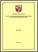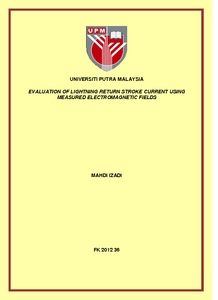Simple Search:

# Evaluation of lightning return stroke current using measured electromagnetic fields

## Citation

Mahdi, Izadi (2012) Evaluation of lightning return stroke current using measured electromagnetic fields. PhD thesis, Universiti Putra Malaysia.

## Abstract / Synopsis

Lightning is an essence of natural phenomenon that can affect on the stability of power lines. It can directly strike the conductor lines, towers, or shielding wires (direct effect). Likewise, the induced over voltages can be generated on the lines, when the lightning strikes the ground surface or any tall object around power lines; so that the electromagnetic fields associated with the lightning channel are coupled with the line conductors (indirect effect). Several studies have been completed to evaluate the return stroke current which can be categorized into two groups; i.e. direct measurement of the current and inverse procedure algorithms based on measured electromagnetic fields for the determination of the return stroke current. In the direct measurement method, the current can be measured by setting current coils at the top of towers or by using the artificial triggered lightning technique to measure channel base currents. On the other hand, indirect methods can evaluate the return stroke current using measured fields and they can cover a greater number of lightning occurrences compared to direct measurement. This study seeks to determine the channel base current and current wave shapes at different heights along lightning channel using measured electromagnetic fields (inverse procedure). It also intends to compute the electromagnetic fields associated with the same lightning channel at the other observation points (direct procedure) to validate the predicted current wave shapes using measured electromagnetic fields, while the ground conductivity is assumed to be perfect. The first section of this study reviews and discusses the most important channel base current functions and current models and electromagnetic calculation methods. In the next sections, this study proposes the field expressions by 2nd FDTD method in order to compute the electromagnetic fields due to realistic current functions in the time domain. For the generalization and linearization of field expressions, the general field equations are proposed directly in the time domain while supporting the most important channel base current functions directly needless of an extra conversion to the other domain. Consequently, the proposed electromagnetic calculation methods can be corroborated with the measured fields and the results from previous studies. Moreover, the field expressions due to inclined lightning channel are proposed and substantiated with the measured fields directly in the time domain, while a realistic channel base current function and the general form of engineering current model are applied. This research proposed an inverse procedure algorithm using the proposed general fields’ expressions and the particle swarm optimization algorithm (PSO) in the time domain where the full channel base current wave shape in time domain can be determined. By considering the three electromagnetic fields components, this method can be applied to all distances from the lightning channel without any limitation. Moreover, in the proposed method, the attenuation height dependent factor can be determined using measured field while assuming it to be an unknown function based on the engineering model. The proposed method can predict the current wave shapes at different heights along the lightning channel and also support the most important general forms of channel base current functions. However, the improvement of Deindorfer and Uman on the Heidler function with an unknown variable is selected as the general channel base current function. Moreover, the current wave shapes are validated with measured field at other observation point when the determined current parameters and the proposed direct procedure algorithms are applied. It should be noted that the measured fields’ data are obtained from the rocket-triggered lightning at two different radial distances from the lightning channel. The proposed algorithm is applied to the measured electric fields’ data from the natural lightning channel while the radial distance is determined using the lightning location system and the simulated electric field using predicted current parameters which are then compared with the measured electric field.Preview
PDF
FK 2012 36R.pdfView Item